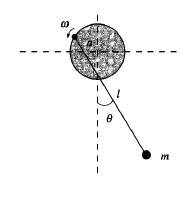# Lagrangian mechanics, simple pendulum

## Homework Statement

A simple pendulum of length ξ and mass m is suspended from a point on the circumference of a thin massless disc of radius α that rotates with a constant angular velocity ω about its central axis as shown in Figure. Find the equation of motion of the mass m.L = T - V

## The Attempt at a Solution

Firstly, I know I should find its x' and y' which representing its velocity.
i.e. T - V = 1/2 m ( x_dot 2 + y_dot 2 ) - mgy

However, I don't know where I should start with to find their x and y.

Thanks.

## The Attempt at a Solution

Firstly, I know I should find its x' and y' which representing its velocity.
i.e. T - V = 1/2 m ( x_dot 2 + y_dot 2 ) - mgy

However, I don't know where I should start with to find their x and y.

Thanks.
first task would be to define the generalized coordinates q and velocities qdots and then proceed for defining the T and V.
your disk is in which plane and which is axis of rotation of the disk.

first task would be to define the generalized coordinates q and qdots and then proceed for defining the T and V.

But how can I define the generalized coordinates q and qdots?

But how can I define the generalized coordinates q and qdots?

define usual coordinates as degrees of freedom permits -write any constraining equations...which are relations between coordinates or velocities.
then the gen. coordinates can be defined.

But how can I define the generalized coordinates q and qdots?

your pendulum is hanging from a disk- so it will be at length L from the disk suppose you place your origin of coordinates at the centre of disk
and the axes X,Y,Z so the bob will lie at -z,x,y but as disl starts rotating the bob will start rotating in a circle and that circle will be a raised one , so at any instant the bob will be at x',y'z' .
the equation of constraint can be that sum of the squares of three coordinates will be equal to length square + rad of the disk squared.
if you choose an angle made by the thread with vertical the cosine of the angle will be z' /L.... similarly other relations can follow and your degrees of freedom will be reduced- the motion may be described by one angle and its time rate of change.

your pendulum is hanging from a disk- so it will be at length L from the disk suppose you place your origin of coordinates at the centre of disk
and the axes X,Y,Z so the bob will lie at -z,x,y but as disl starts rotating the bob will start rotating in a circle and that circle will be a raised one , so at any instant the bob will be at x',y'z' .
the equation of constraint can be that sum of the squares of three coordinates will be equal to length square + rad of the disk squared.
if you choose an angle made by the thread with vertical the cosine of the angle will be z' /L.... similarly other relations can follow and your degrees of freedom will be reduced- the motion may be described by one angle and its time rate of change.

I try to begin the question with place origin of coordinates at the centre of the disk (x,y)
With the parameters of figures given,
for x, because ξsinθ ( the height of triangle formed by bob ) is longer than that of radius,
x = ξsinθ - a cos ωt
similarly, y = ξcosθ - a sin ωt

However, the answer is x = a cos ωt + ξ sin θ, y = a sin ωt - ξcosθ
Am I missing something?

If I just begin the problem that the disk rotates on the other side, I get the same answer as textbook.
Last night, I spent a night to watch youtube mechanics and vector teaching videos and read over my textbook but still I could not get the answer.
I am new to Mechanics. sorry for any annoying questions :'(